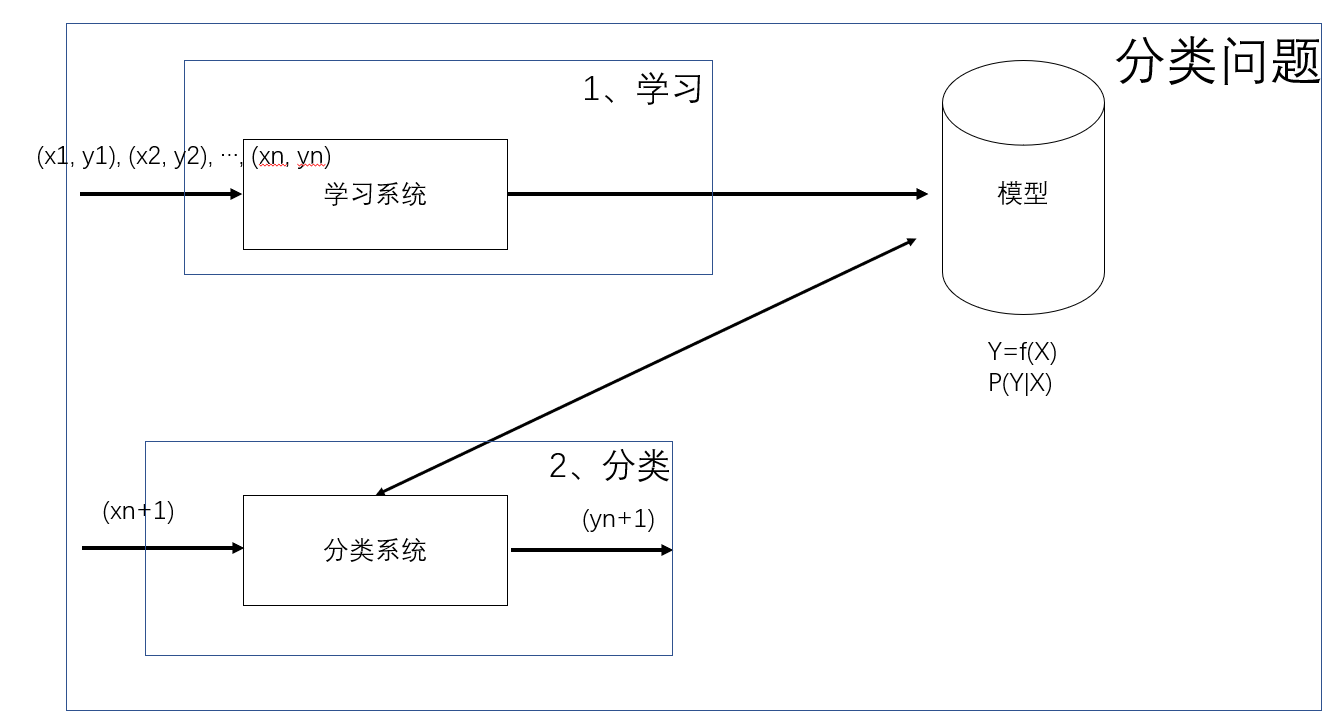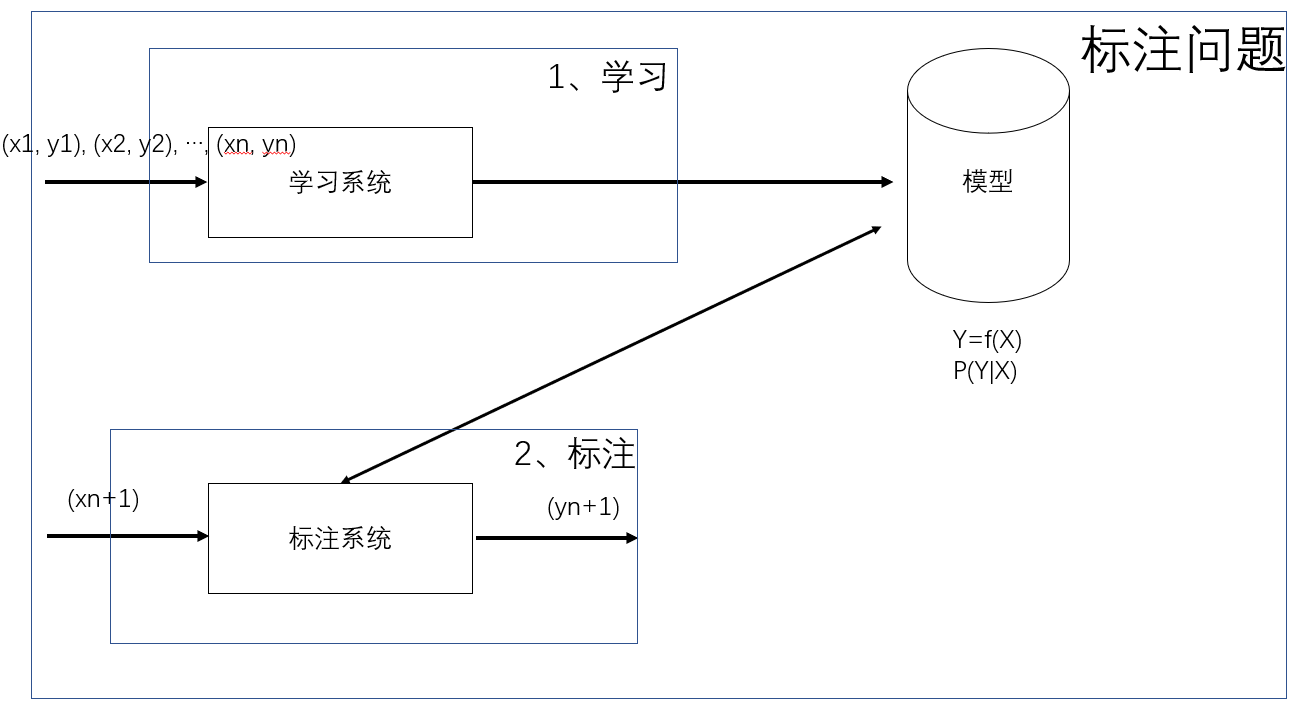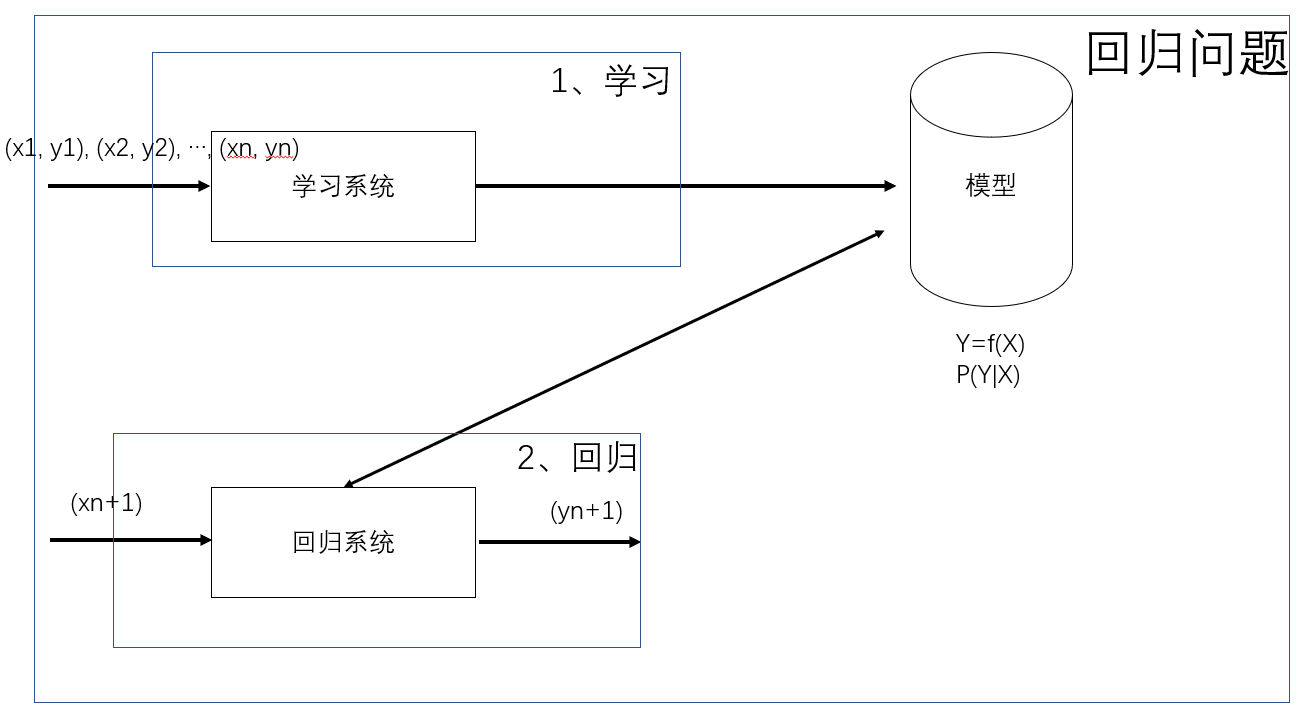# 统计机器学习【1】- 入门机器学习（二）

## 一、分类问题$TP——将正类预测为正类数；$

$FN——将正类预测为负类数；$

$FP——将负类预测为正类数；$

$TN——将负类预测为负类数；$

$P = \frac{TP}{TP + FP}$

$R = \frac{TP}{TP + FN}$

$\frac{2}{F_1} = \frac{1}{P} + \frac{1}{R}$
$F_1 = \frac{2TP}{2TP + FP + FN}$

## 二、标注问题## 三、回归问题（1）输入变量个数：

• 一元回归
• 多元回归
（2）模型类型：
• 线性回归
• 非线性回归

【1】统计学习方法-李航©️2019 CSDN 皮肤主题: 游动-白 设计师: 上身试试点击重新获取扫码支付